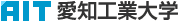日本語
 Please use this identifier to cite or link to this item: `http://hdl.handle.net/11133/579`

 Title: コンクリートの圧縮強度と引張強度の確率分布と寸法効果に関する研究 Other Titles: コンクリート ノ アッシュク キョウド ト インチョウ キョウド ノ カクリツ ブンプ ト スンポウ コウカ ニカンスル ケンキュウStudy on Probability Distribution and Size Effect of Compressive and Tensile Strength of Concrete Authors: 小池, 狹千朗加藤, 善之助KOIKE, SachioKATO, Zennosuke Issue Date: 31-Mar-1981 Publisher: 愛知工業大学 Abstract: In the case of calculating the probability distribution of strength of reinforced concrete members by Monte Carlo simulation by the computer, it is very important to sample the probability distribution of mechanical properties of cach materials. This study examined the probability distribution and size effect of compressive strength of three kinds of concrete cylinders and four kinds of concrete prisms and direct tensile strength of four kinds of concrete prisms and splitting tensile strength of three kinds of concrete cylinders, using four kinds of concrete mix proportions having each different maximum sizes of aggregates. The experimental value of strength shows the probability distribution quite close to the straight line when plotted either on weibull probability paper or on normal ones, but some values are slightly apart from the straight line near the maximum and minimum experimental values. But, experimental values of coefficient of variation CV of strength of concrete show the lower values than theoretical ones indicated by the formula CV=√<Γ(1+2/β+1)/Γ^2(1+1/β+1)-1>, wcere, β is parameter related to properties of concrete and Γ is gamma function. Therefore, the probability distribution of its strength cannot always be expressed by the weibull distribution. Compressive strength of concrete decreases with decrease in size of specimen, both in prism and cylinder specimen, and with increase in size of aggregate. On the other hands, tensile strength of concrete reaches the top at specimen size of 10cm both in prism and cylinder specimens, but it rather decreases when specimen size becomes smaller than 10cm. URI: http://hdl.handle.net/11133/579 Appears in Collections: 16号

Files in This Item:

File Description SizeFormatDSpace Software Copyright © 2002-2010  Duraspace - Feedback